Processing ......FreeComputerBooks.com Links to Free Computer, Mathematics, Technical Books all over the World

Discrete Mathematics for Computer Science Students
Top Free Computer Networking Books 🌠 - 100% Free or Open Source!
• Title Discrete Mathematics for Computer Science Students
• Author(s) Kenneth P. Bogart
• Publisher: Dartmouth College (2003)
• Hardcover/Paperback N/A
• eBook PDF Files
• Language: English
• ISBN-10/ASIN: 0132122715
• ISBN-13: N/A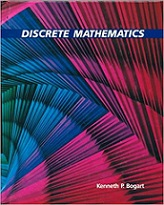Book Description

This book gives thorough coverage to topics that have great importance to computer scientists and provides a motivating computer science example for each math topic. Contents: Counting; Cryptography and Number Theory; Reflections on Logic and Proof.

With a clear presentation of essential concepts, it provides a lucidly written introduction to discrete mathematics with abundant support for learning, and demonstrates a wide range of applications relevant to computer science majors.

• Kenneth P. Bogart was an American mathematician known for his work on preference structures and for his textbook on combinatorics. He was a professor at Dartmouth College. Before his death in 2005, Ken Bogart was in the final stages of completing an NSF-sponsored project about the teaching of Combinatorics Through Guided Discovery.
Reviews, Ratings, and Recommendations: Related Book Categories: Read and Download Links:Similar Books:
•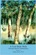A Cool Brisk Walk Through Discrete Mathematics (Stephen Davies)

This is a completely and forever free and open source educational materials dedicated to the mathematics that budding computer science practitioners actually need to know. They feature the fun and addictive teaching of award-winning lecturer!

•Mathematics for Computer Science (Eric Lehman, et al)

This book covers elementary discrete mathematics for computer science and engineering. It emphasizes mathematical definitions and proofs as well as applicable methods. explores the topics of basic combinatorics, number and graph theory, logic and proof techniques.

•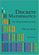Discrete Mathematics: An Open Introduction (Oscar Levin)

This is a gentle introduction to discrete mathematics. Four main topics are covered: counting, sequences, logic, and graph theory. Along the way proofs are introduced, including proofs by contradiction, proofs by induction, and combinatorial proofs.

•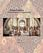Isomorphism - Mathematics of Programming (Xinyu Liu)

This book introduces the mathematics behind computer programming. It intents to tell: programming is isomorphic to mathematics. Just like in art and music, there are interesting stories and mathematicians behind the great minds.

•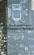Applied Discrete Structures, Fundamentals (Al Doerr, et al)

This book contains most of the fundamental concepts taught in a one semester course in discrete mathematics which is a required course for students in Computer Science, Mathematics and Information Technology.

•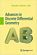Advances in Discrete Differential Geometry (Alexander I. Bobenko)

It surveys the fascinating connections between discrete models in differential geometry and complex analysis, integrable systems and applications in computer graphics and is illustrated to convince readers it's both beautiful and useful.

•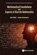Mathematical Foundations and Aspects of Discrete Mathematics

This is a book about discrete mathematics which also discusses mathematical reasoning and logic. It offers an introduction to discrete mathematics for undergraduate students in computer science and mathematics.

•A Spiral Workbook for Discrete Mathematics (Harris Kwong)

This is a book on discrete mathematics with an emphasis on motivation. It explains and clarifies the unwritten conventions in mathematics, and guides the students through a detailed discussion on how a proof is revised from its draft to a final polished form.

•Discrete Structures for Computer Science: Counting, Recursion

This book provides a broad introduction to some of the most fascinating and beautiful areas of discrete mathematical structures with examples in logic, applications of the principle of inclusion and exclusion and finally the pigeonhole principal.

•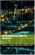Mathematics for Computer Scientists (Gareth J. Janacek, et al)

The aim of this book is to present some the basic mathematics that is needed by computer scientists. The reader is not expected to be a mathematician and we hope will find what follows useful.

•Lists, Decisions and Graphs - With an Introduction to Probability

In this book, four basic areas of discrete mathematics are presented: Counting and Listing (Unit CL), Functions (Unit Fn), Decision Trees and Recursion (Unit DT), and Basic Concepts in Graph Theory (Unit GT).

Book Categories
 :All CategoriesTop Free BooksRecent BooksMiscellaneous BooksComputer EngineeringComputer LanguagesComputer ScienceData Science/DatabasesJava and Java EE (J2EE)Linux and UnixMathematicsMicrosoft and .NETMobile ComputingNetworking and CommunicationsSoftware EngineeringSpecial TopicsWeb Programming
Other Categories